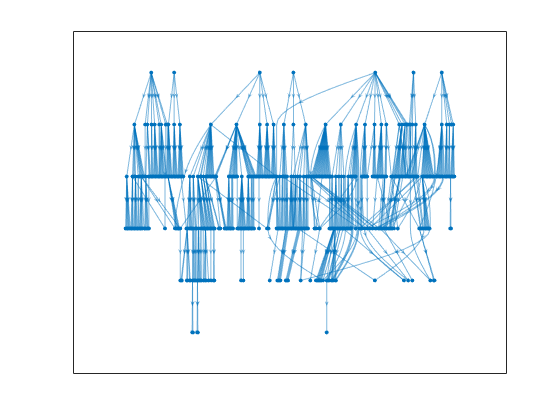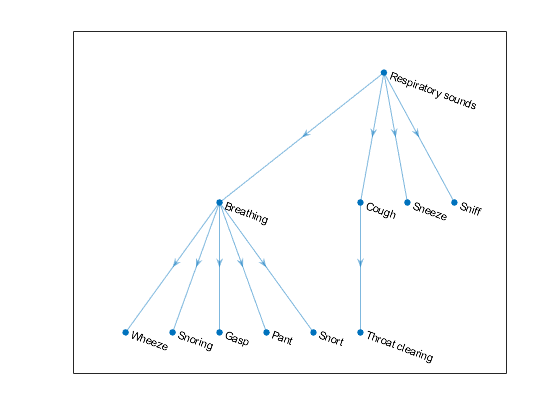# yamnetPreprocess

Preprocess audio for YAMNet classification

## Syntax

``features = yamnetPreprocess(audioIn,fs)``
``features = yamnetPreprocess(audioIn,fs,'OverlapPercentage',OP)``

## Description

example

````features = yamnetPreprocess(audioIn,fs)` generates mel spectrograms from `audioIn` that can be fed to the YAMNet pretrained network.```
````features = yamnetPreprocess(audioIn,fs,'OverlapPercentage',OP)` specifies the overlap percentage between consecutive audio frames.For example, ```features = yamnetPreprocess(audioIn,fs,'OverlapPercentage',75)``` applies a 75% overlap between consecutive frames used to generate the spectrograms.```

## Examples

collapse all

Type `yamnet` at the Command Window. If the Audio Toolbox model for YAMNet is not installed, then the function provides a link to the location of the network weights. To download the model, click the link. Unzip the file to a location on the MATLAB path.

```downloadFolder = fullfile(tempdir,'YAMNetDownload'); loc = websave(downloadFolder,'https://ssd.mathworks.com/supportfiles/audio/yamnet.zip'); YAMNetLocation = tempdir; unzip(loc,YAMNetLocation) addpath(fullfile(YAMNetLocation,'yamnet'))```

Check that the installation is successful by typing `yamnet` at the Command Window. If the network is installed, then the function returns a `SeriesNetwork` (Deep Learning Toolbox) object.

`yamnet`
```ans = SeriesNetwork with properties: Layers: [86×1 nnet.cnn.layer.Layer] InputNames: {'input_1'} OutputNames: {'Sound'} ```

Load a pretrained YAMNet convolutional neural network and examine the layers and classes.

Use `yamnet` to load the pretrained YAMNet network. The output net is a `SeriesNetwork` (Deep Learning Toolbox) object.

`net = yamnet`
```net = SeriesNetwork with properties: Layers: [86×1 nnet.cnn.layer.Layer] InputNames: {'input_1'} OutputNames: {'Sound'} ```

View the network architecture using the `Layers` property. The network has 86 layers. There are 28 layers with learnable weights: 27 convolutional layers, and 1 fully connected layer.

`net.Layers`
```ans = 86x1 Layer array with layers: 1 'input_1' Image Input 96×64×1 images 2 'conv2d' Convolution 32 3×3×1 convolutions with stride [2 2] and padding 'same' 3 'b' Batch Normalization Batch normalization with 32 channels 4 'activation' ReLU ReLU 5 'depthwise_conv2d' Grouped Convolution 32 groups of 1 3×3×1 convolutions with stride [1 1] and padding 'same' 6 'L11' Batch Normalization Batch normalization with 32 channels 7 'activation_1' ReLU ReLU 8 'conv2d_1' Convolution 64 1×1×32 convolutions with stride [1 1] and padding 'same' 9 'L12' Batch Normalization Batch normalization with 64 channels 10 'activation_2' ReLU ReLU 11 'depthwise_conv2d_1' Grouped Convolution 64 groups of 1 3×3×1 convolutions with stride [2 2] and padding 'same' 12 'L21' Batch Normalization Batch normalization with 64 channels 13 'activation_3' ReLU ReLU 14 'conv2d_2' Convolution 128 1×1×64 convolutions with stride [1 1] and padding 'same' 15 'L22' Batch Normalization Batch normalization with 128 channels 16 'activation_4' ReLU ReLU 17 'depthwise_conv2d_2' Grouped Convolution 128 groups of 1 3×3×1 convolutions with stride [1 1] and padding 'same' 18 'L31' Batch Normalization Batch normalization with 128 channels 19 'activation_5' ReLU ReLU 20 'conv2d_3' Convolution 128 1×1×128 convolutions with stride [1 1] and padding 'same' 21 'L32' Batch Normalization Batch normalization with 128 channels 22 'activation_6' ReLU ReLU 23 'depthwise_conv2d_3' Grouped Convolution 128 groups of 1 3×3×1 convolutions with stride [2 2] and padding 'same' 24 'L41' Batch Normalization Batch normalization with 128 channels 25 'activation_7' ReLU ReLU 26 'conv2d_4' Convolution 256 1×1×128 convolutions with stride [1 1] and padding 'same' 27 'L42' Batch Normalization Batch normalization with 256 channels 28 'activation_8' ReLU ReLU 29 'depthwise_conv2d_4' Grouped Convolution 256 groups of 1 3×3×1 convolutions with stride [1 1] and padding 'same' 30 'L51' Batch Normalization Batch normalization with 256 channels 31 'activation_9' ReLU ReLU 32 'conv2d_5' Convolution 256 1×1×256 convolutions with stride [1 1] and padding 'same' 33 'L52' Batch Normalization Batch normalization with 256 channels 34 'activation_10' ReLU ReLU 35 'depthwise_conv2d_5' Grouped Convolution 256 groups of 1 3×3×1 convolutions with stride [2 2] and padding 'same' 36 'L61' Batch Normalization Batch normalization with 256 channels 37 'activation_11' ReLU ReLU 38 'conv2d_6' Convolution 512 1×1×256 convolutions with stride [1 1] and padding 'same' 39 'L62' Batch Normalization Batch normalization with 512 channels 40 'activation_12' ReLU ReLU 41 'depthwise_conv2d_6' Grouped Convolution 512 groups of 1 3×3×1 convolutions with stride [1 1] and padding 'same' 42 'L71' Batch Normalization Batch normalization with 512 channels 43 'activation_13' ReLU ReLU 44 'conv2d_7' Convolution 512 1×1×512 convolutions with stride [1 1] and padding 'same' 45 'L72' Batch Normalization Batch normalization with 512 channels 46 'activation_14' ReLU ReLU 47 'depthwise_conv2d_7' Grouped Convolution 512 groups of 1 3×3×1 convolutions with stride [1 1] and padding 'same' 48 'L81' Batch Normalization Batch normalization with 512 channels 49 'activation_15' ReLU ReLU 50 'conv2d_8' Convolution 512 1×1×512 convolutions with stride [1 1] and padding 'same' 51 'L82' Batch Normalization Batch normalization with 512 channels 52 'activation_16' ReLU ReLU 53 'depthwise_conv2d_8' Grouped Convolution 512 groups of 1 3×3×1 convolutions with stride [1 1] and padding 'same' 54 'L91' Batch Normalization Batch normalization with 512 channels 55 'activation_17' ReLU ReLU 56 'conv2d_9' Convolution 512 1×1×512 convolutions with stride [1 1] and padding 'same' 57 'L92' Batch Normalization Batch normalization with 512 channels 58 'activation_18' ReLU ReLU 59 'depthwise_conv2d_9' Grouped Convolution 512 groups of 1 3×3×1 convolutions with stride [1 1] and padding 'same' 60 'L101' Batch Normalization Batch normalization with 512 channels 61 'activation_19' ReLU ReLU 62 'conv2d_10' Convolution 512 1×1×512 convolutions with stride [1 1] and padding 'same' 63 'L102' Batch Normalization Batch normalization with 512 channels 64 'activation_20' ReLU ReLU 65 'depthwise_conv2d_10' Grouped Convolution 512 groups of 1 3×3×1 convolutions with stride [1 1] and padding 'same' 66 'L111' Batch Normalization Batch normalization with 512 channels 67 'activation_21' ReLU ReLU 68 'conv2d_11' Convolution 512 1×1×512 convolutions with stride [1 1] and padding 'same' 69 'L112' Batch Normalization Batch normalization with 512 channels 70 'activation_22' ReLU ReLU 71 'depthwise_conv2d_11' Grouped Convolution 512 groups of 1 3×3×1 convolutions with stride [2 2] and padding 'same' 72 'L121' Batch Normalization Batch normalization with 512 channels 73 'activation_23' ReLU ReLU 74 'conv2d_12' Convolution 1024 1×1×512 convolutions with stride [1 1] and padding 'same' 75 'L122' Batch Normalization Batch normalization with 1024 channels 76 'activation_24' ReLU ReLU 77 'depthwise_conv2d_12' Grouped Convolution 1024 groups of 1 3×3×1 convolutions with stride [1 1] and padding 'same' 78 'L131' Batch Normalization Batch normalization with 1024 channels 79 'activation_25' ReLU ReLU 80 'conv2d_13' Convolution 1024 1×1×1024 convolutions with stride [1 1] and padding 'same' 81 'L132' Batch Normalization Batch normalization with 1024 channels 82 'activation_26' ReLU ReLU 83 'global_average_pooling2d' Global Average Pooling Global average pooling 84 'dense' Fully Connected 521 fully connected layer 85 'softmax' Softmax softmax 86 'Sound' Classification Output crossentropyex with 'Speech' and 520 other classes ```

To view the names of the classes learned by the network, you can view the `Classes` property of the classification output layer (the final layer). View the first 10 classes by specifying the first 10 elements.

`net.Layers(end).Classes(1:10)`
```ans = 10×1 categorical Speech Child speech, kid speaking Conversation Narration, monologue Babbling Speech synthesizer Shout Bellow Whoop Yell ```

Use `analyzeNetwork` (Deep Learning Toolbox) to visually explore the network.

`analyzeNetwork(net)`YAMNet was released with a corresponding sound class ontology, which you can explore using the `yamnetGraph` object.

```ygraph = yamnetGraph; p = plot(ygraph); layout(p,'layered')```The ontology graph plots all 521 possible sound classes. Plot a subgraph of the sounds related to respiratory sounds.

```allRespiratorySounds = dfsearch(ygraph,"Respiratory sounds"); ygraphSpeech = subgraph(ygraph,allRespiratorySounds); plot(ygraphSpeech)````[audioIn,fs] = audioread('SpeechDFT-16-8-mono-5secs.wav');`

Plot and listen to the audio signal.

```T = 1/fs; t = 0:T:(length(audioIn)*T) - T; plot(t,audioIn); grid on xlabel('Time (t)') ylabel('Ampltiude')````soundsc(audioIn,fs)`

Use `yamnetPreprocess` to extract mel spectrograms from the audio signal. Visualize an arbitrary spectrogram from the array.

```melSpectYam = yamnetPreprocess(audioIn,fs); arbSpect = melSpectYam(:,:,1,randi(size(melSpectYam,4))); surf(arbSpect,'EdgeColor','none') view([90,-90]) axis([1 size(arbSpect,1) 1 size(arbSpect,2)]) xlabel('Mel Band') ylabel('Frame') title('Mel Spectrogram for YAMNet') axis tight```Create a YAMNet neural network (This requires Deep Learning Toolbox). Call `classify` with your YAMNet network and the preprocessed mel spectrogram images.

```net = yamnet; classes = classify(net,melSpectYam);```

Classify the audio signal as the most frequently occurring sound.

`mySound = mode(classes)`
```mySound = categorical Speech ```

## Input Arguments

collapse all

Input signal, specified as a column vector or matrix. If you specify a matrix, `yamnetPreprocess` treats the columns of the matrix as individual audio channels.

Data Types: `single` | `double`

Sample rate of the input signal in Hz, specified as a positive scalar.

Data Types: `single` | `double`

Percentage overlap between consecutive mel spectrograms, specified as the comma-separated pair consisting of `'OverlapPercentage'` and a scalar in the range [0,100).

Data Types: `single` | `double`

## Output Arguments

collapse all

Mel spectrograms generated from `audioIn`, returned as a `96`-by-`64`-by-`1`-by-K array, where:

• `96` –– Represents the number of 10 ms frames in each mel spectrogram

• `64` –– Represents the number of mel bands spanning 125 Hz to 7.5 kHz

• K –– Represents the number of mel spectrograms and depends on the length of `audioIn`, the number of channels in `audioIn`, as well as `OverlapPercentage`

Note

Each `96`-by-`64`-by-`1` patch represents a single mel spectrogram image. For multichannel inputs, mel spectrograms are stacked along the fourth dimension.

Data Types: `single`

 Gemmeke, Jort F., et al. “Audio Set: An Ontology and Human-Labeled Dataset for Audio Events.” 2017 IEEE International Conference on Acoustics, Speech and Signal Processing (ICASSP), IEEE, 2017, pp. 776–80. DOI.org (Crossref), doi:10.1109/ICASSP.2017.7952261.

 Hershey, Shawn, et al. “CNN Architectures for Large-Scale Audio Classification.” 2017 IEEE International Conference on Acoustics, Speech and Signal Processing (ICASSP), IEEE, 2017, pp. 131–35. DOI.org (Crossref), doi:10.1109/ICASSP.2017.7952132.

## Support#### Try MATLAB, Simulink, and Other Products

Get trial now